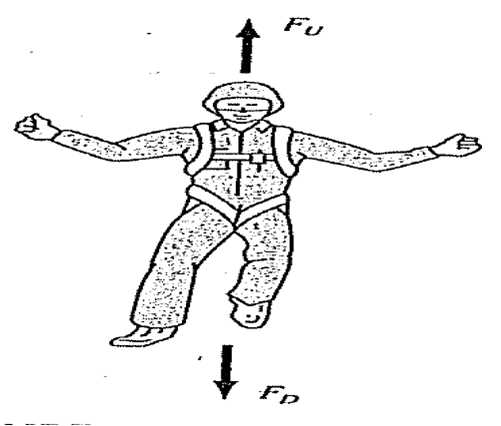help: euler() prg on home mode
05-30-2017, 02:36 PM (This post was last modified: 06-07-2017 02:59 PM by compsystems.)
Post: #1compsystemsSenior Member Posts: 1,343 Joined: Dec 2013
help: euler() prg on home mode
Hello, the next numerical code, I want to run it in HOME MODE.

The definition v(t) does not allow me to define it within the code, Why?

PHP Code:
// file euler.hppprgmexport euler()BEGIN    local g,c,m,t;    local vi,ti,vf,tf,iteration;    //local v;    purge(g,c,m,t,vi,ti,vf,tf);    v(t):= vi+(g-(c/m)*vi)*(t-ti);    g:=9.81; c:=12.5; m:=68.1;    vi:=0;    ti:=0;    iteration:=1;    print; // clear terminal view (output)        print("euler");    print("press [↓] for next iteration" );    print("press [on] for exit" );    wait(1);       FOR tf FROM 0 TO 10 STEP 2 DO        print; // clear terminal view (output)        print("iteration = "+iteration);        vf:=v(tf);        print("vi = "+vi+" m/s");        print("ti = "+ti+" s");        print("tf = "+tf+" s");        print("vf("+tf+") = "+vf+" m/s");        print("");        vi:=vf;        ti:=tf;        iteration:=iteration+1;         wait();    END;    print("Iterations finished," );    return("Done");END;
Source: NUMERICAL METHODS FOR ENGINEERS Steven C. Chapra & Raymond P. CanaleI managed to run it by doing the following trick.
Icomment the line // v(t):= vi+(g-(c/m)*vi)*(t-ti);
and I put in the line of entry (CAS MODE) the expression
v(t):= vi + (g- (c / m) * vi) * (t-ti);

euler() returns [] =(
but
[shift](program editor) euler
[DEBUG][CONT] [enter] ...[enter] ... ok
The program shows me screen by screen what I want
vi=0 m/s, ti=0 s , tf=0 s
v(0) = 0 m/s

vi=0, ti=0, tf=2
v(2) = 19.62 m/s

vi=19.62 m/s, ti=2 s, tf=4 s
v(4) = 32.04 m/s

vi=32.04 m/s, ti=4 s, tf=6 s
v(6") = 39.90 m/s
...

Please someone can help me by rewriting the above code to work well

Thanks

PS: In CAS mode if it works fine but how can I clean each screen? The print; command does not seem to be working. I have to clean each screen because the terminal view does not progress after the first impression screen is completed, (even hp-prime is a single process) should allow split screen and more of a process as did the hp48,

PHP Code:
// file euler1.hppprgm// version simple MODO CAS#cas  euler1():=  BEGIN    local g,c,m,t;    local vi,ti,vf,tf,iteration;    local v;    purge(g,c,m,t,vi,ti,vf,tf);    v(t):= vi+(g-(c/m)*vi)*(t-ti);    g:=9.81; c:=12.5; m:=68.1;    vi:=0;    ti:=0;    iteration:=1;    PRINT(); // clear terminal view (output)    print("euler1");    print("press [↓] for next iteration" );    print("press [on] for exit" );    wait(1);          FOR tf FROM 0 TO 10 STEP 2 DO      PRINT(); // clear terminal view (output)      print("iteration = "+iteration);      vf:=v(tf);      print("vi = "+vi+" m/s");      print("ti = "+ti+" s");      print("tf = "+tf+" s");      print("vf("+tf+") = "+vf+" m/s");      print("");      vi:=vf;      ti:=tf;      iteration:=iteration+1;       wait();    END;    print("Iterations finished" );    return("Done");  END;#end

Debug mode does not work for CAS MODE = (, for this reason I want to create it in HOME MODE
 « Next Oldest | Next Newest »

 Messages In This Thread help: euler() prg on home mode - compsystems - 05-30-2017 02:36 PM RE: help: euler() prg on home mode - toml_12953 - 05-30-2017, 03:59 PM RE: help: euler() prg on home mode - compsystems - 05-30-2017, 04:18 PM RE: help: euler() prg on home mode - toml_12953 - 05-30-2017, 05:03 PM RE: help: euler() prg on home mode - compsystems - 05-30-2017, 05:17 PM